# Matrices

The word matrix refers to a rectangular array of elements. Matrices have utility in procedures for transforming such sets of elements. For example, one type of procedure would represent the transformation from one set of coordinate axes to another. Another is the solution of linear sets of equations.

Common notation for matrices uses a boldface letter for a matrix and identifies its elements in terms of the rows and columns of the array. The elements are typically specified by subscripts arc with the row subscript first.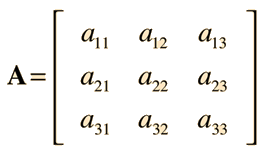A shorthand notation for matrices is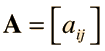Matrices of the same dimensions can be added, subtracted, or multiplied by a constant in the same way as ordinary numbers by applying the operation to each element. These operations follow combination rules similar to ordinary numbers.

Given that a matrix is a rectangular array, we may speak of an m x n matrix (read m by n matrix) as one which has m rows and n columns.

Using the shorthand notation for matrices introduced above, we can describe some of the properties of matrices. The transpose AT of an m x n matrix A[ajk] is the n x m matrix that has the first row of A as its first column, the second row of A as its second column, and so on. Symmetric matrices and skew-symmetric matrices are square matrices whose transpose equals the matrix or minus the matrix, respectively: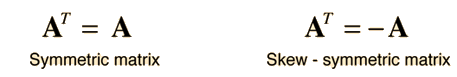Matrix multiplication requires a defined procedure and is defined for two matrices only if the number of rows of the second matrix is equal to the number of columns of the first as will be shown below.

 Matrix Multiplication
Index

Reference

Kreysig
Ch 6

 HyperPhysics****HyperMath*****Linear Algebra R Nave
Go Back

# Matrix Multiplication

The applications of matrices often involve the multiplication of two matrices, which requires rules for combination of the elements of the matrices. Using single bold capital letters to represent matrices, the multiplication can be written: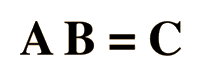Using the standard practice of using lower case letters for the elements of the matrices, with two subscripts in the order row and column, this matrix multiplication process for 3x3 matrices can be depicted as: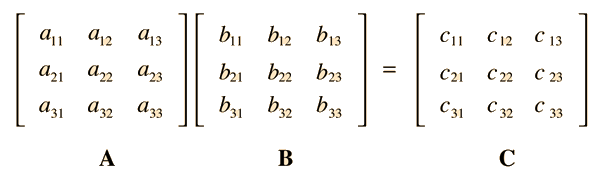Multiplying the matrices involves finding the elements cij of the product matrix by applying a specific rule, which involves multiplying the elements of the ith row of matrix A by the elements of the jth column of matrix B. This is confusing enough that it may help to depict the process visually as follows: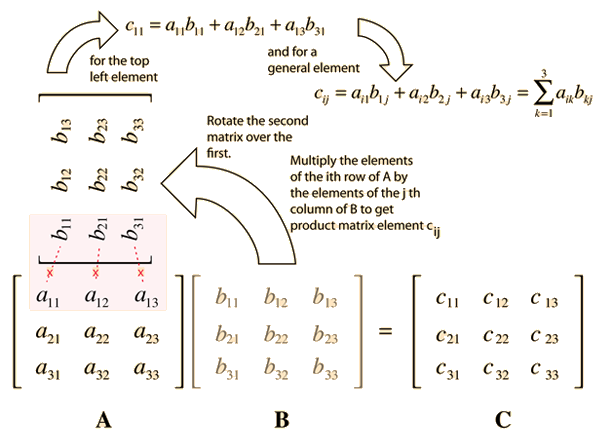The operations that are done in calculating the product of two matrices are the same as those done in forming a scalar product of two vectors. It might be helpful to think of the process as forming element cjk by taking the scalar product of row j of A and column k of B.

Given the nature of the matrix product, it is defined only if the number of rows in matrix B is the same as the number of columns of matrix A. When the matrix product AB is formed, the product matrix will have the same number of rows as A and the same number of columns as B as illustrated below.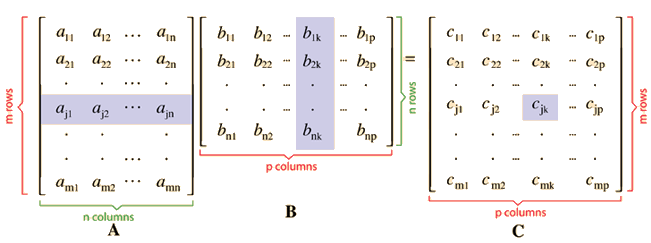The shaded areas are a reminder that the jth row of matrix A and the kth column of matrix B are combined to produce the value of the coefficient cjk in the product matrix C.

Index

Kreysig
Ch 6

 HyperPhysics****HyperMath*****Linear Algebra R Nave
Go Back

# Matrix Combination Rules

For addition and multiplication by a constant, matrices follow combination rules similar to those in algebra. Notable differences appear in matrix multiplication.

A + B = B + A
A + (B+C) = (A+B) + C
A + 0 = A

Scalar multiplication (c and k are scalars):

c(A + B) = cB + cA
(c + k)A = cA + kA
c(kA = (ck)A
1A =A

Matrix multiplication has some distinctly different properties:

AB ≠ BA in general.
AB = 0 does not imply A = 0 or B = 0 or BA = 0
AC = AD does not imply C = D

Matrix multiplication has some properties similar to those for numbers (k = scalar):

(kA)B = k(AB) = A (kB)
A(BC) = (AB)C
(A + B)C = AC + BC
Index

Kreysig
Ch 6

 HyperPhysics****HyperMath*****Linear Algebra R Nave
Go Back